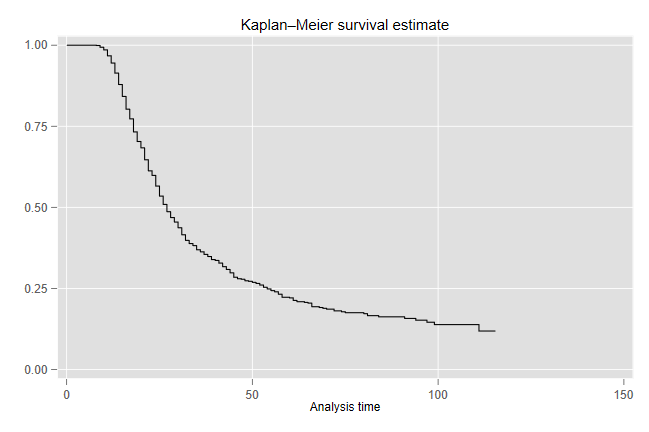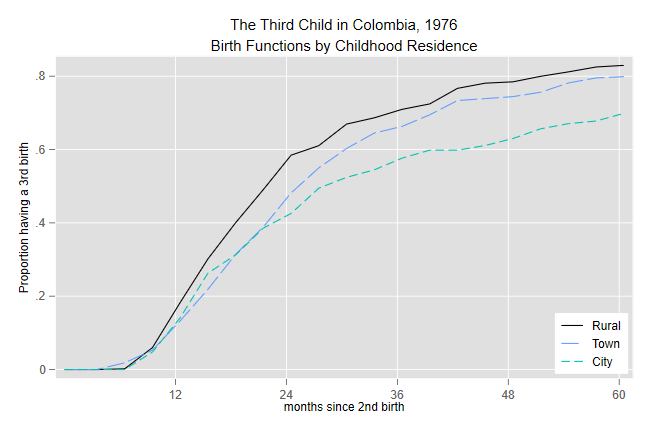Germán Rodríguez
Demographic Methods Princeton University## The Third Child in Colombia

We will look at the interval from second to third birth using Colombian WFS data, circa 1976. We start from an extract that has the dates of R’s birth, date of interview, the birth history, date of first union if any, and current and childhood type of place of residence.

```. use https://grodri.github.io/datasets/cofertx, clear
(COSR02 extract)
```

#### Time at Risk and Event Indicator

We will use intervals that start in the ten years before the interview and exclude twins. (The results are a bit different from my paper with Hobcraft, which used all birth intervals, but I can reproduce the earlier results by removing the period restriction.)

```. keep if b022 >= v007-120 & b022 < v007
(4,144 observations deleted)

. drop if b032==b022      // only 6
(6 observations deleted)
```

We construct time at risk starting in the middle of the month of birth of the second child and ending in the middle of the month when the third child is born or at the end of the month before the interview, whichever occurs first

```. gen expo = b032 - b022

. replace expo = v007 - b022 -0.5 if v007 <= b032

. gen third = b032 < v007
```

If we `stset` the data we can take advantage of Stata’s survival analysis facilities. For example it is very easy to plot a Kaplan-Meier estimate of survival at parity 2:

```. gen id=_n

. stset expo, fail(third) id(id)

Survival-time data settings

ID variable: id
Failure event: third!=0 & third<.
Observed time interval: (expo[_n-1], expo]
Exit on or before: failure

──────────────────────────────────────────────────────────────────────────
1,228  total observations
0  exclusions
──────────────────────────────────────────────────────────────────────────
1,228  observations remaining, representing
1,228  subjects
732  failures in single-failure-per-subject data
34,388  total analysis time at risk and under observation
At risk from t =         0
Earliest observed entry t =         0
Last observed exit t =     115.5

. sts graph

Failure _d: third
Analysis time _t: expo
ID variable: id

. graph export co3rdkm.png, replace
file co3rdkm.png saved as PNG format
```#### Segments of Exposure

We will take advantage of Stata’s facilities to split the exposure into 3 month segments. The cutpoints have the form 0 3.5 6.5 9.5 … 57.5 60.5 120.

```. stsplit segment, at(0 3.5(3)60.5 120)
(9,844 observations (episodes) created)
```

Stata’s built-in variables `_t` and `_t0` have the start and end of each segment and `_d` has the death indicator. We use these to compute events and exposure. This is not a bad time to save the data.

```. replace expo = _t-_t0

. gen births = _d

. save temp,replace
file temp.dta saved
```

We then collapse to obtain total events and exposure by duration (segment) and childhood place of residence.

```. drop if v703 > 3 // a few missing values
(36 observations deleted)

. collapse (sum) births (sum) expo , by(segment v703)
```

At this point we can easily calculate life tables.

#### The Birth Function

I will compute the hazard dividing births by exposure and then use the constant hazard assumption to estimate the cumulative hazard and survival (or rather its complement, the birth function). You can get very similar results assuming that events occur half-way through each three-month interval. (Even more similar if we had used single months.)

```. gen h = births/expo

. bysort v703 (segment): gen H = 3*sum(h)

. gen B = 1-exp(-H)       // the birth function

. separate B, by(v703)

Variable      Storage   Display    Value
name         type    format    label      Variable label
────────────────────────────────────────────────────────────────────────────────
B1              float   %9.0g                 B, v703 == Rural
B2              float   %9.0g                 B, v703 == Town
B3              float   %9.0g                 B, v703 == City

. line B1 B2 B3 segment, lpat(solid longdash dash) ///
>   title("The Third Child in Colombia, 1976") ///
>   subtitle(Birth Functions by Childhood Residence) ///
>   ytitle(Proportion having a 3rd birth) ///
>   xlabel(12 24 36 48 60) xtitle(months since 2nd birth) ///
>   legend(order(1 "Rural" 2 "Town" 3 "City") ///
>   col(1) ring(0) pos(5))

. graph export co3rdbirth.png, replace
file co3rdbirth.png saved as PNG format
```We see that the proportions moving to parity three are higher for women of rural origins than for others at every duration. Women who grew up in towns are a bit less likely to have a third child, and when they do they have it later. Women who grew up in cities are much less likely to have a third child in the long run, but they are also more likely than townfolk to have very short intervals, with a cross-over around 18 months.

#### Quintums and Trimeans

To compute quintums we need to interpolate in the category 57.5-60.5. Linear interpolation would probably do, but with constant hazard a more exact calculation based on S(60) = S(57.5) exp{-2.5 h(57.5)} gives Q = 1-(1-B(57.5))exp{-2.5 h(57.5)}. Note that the way we calculated things, the survival and birth functions pertain to the end of each segment, which is why the code below looks at 54.5

```. gen Q =1-(1-B[_n]) * exp(-2.5*h[_n+1]) if  segment==54.5
(60 missing values generated)

. list v703 segment h B Q if segment > 54 & v703 < 9, sep(3)

┌──────────────────────────────────────────────────┐
│  v703   segment          h          B          Q │
├──────────────────────────────────────────────────┤
19. │ Rural      54.5   .0207612   .8118494   .8230233 │
20. │ Rural      57.5   .0244898   .8251771          . │
21. │ Rural      60.5   .0086022    .829631          . │
├──────────────────────────────────────────────────┤
40. │  Town      54.5   .0363636   .7815775   .7930105 │
41. │  Town      57.5   .0215054   .7952242          . │
42. │  Town      60.5   .0059435   .7988432          . │
├──────────────────────────────────────────────────┤
61. │  City      54.5   .0137931   .6706035   .6766252 │
62. │  City      57.5   .0073801   .6778163          . │
63. │  City      60.5   .0223537   .6987138          . │
└──────────────────────────────────────────────────┘
```

So the quintums are 82.3, 79.3 and 67.7 for women who grew up in rural areas, towns and cities. (These are a bit lower than in my paper with Hobcraft, reflecting the fact that they pertain to more recent births.)

To obtain the trimean we use a similar interpolation strategy for computing the quartiles and then apply Tukey’s formula. I will illustrate with rural women. A quarter of the quintum of .823 is .206, so we need the durations by which 20.6, 41.2 and 61.7% of the women have moved to parity 3. For the first value we need to interpolate in category 9.5-12.5. Write 1 - 0.206 = (1-B(9.5)) exp{-2.5 h(9.5)(Q1-9.5)} and solve for Q1 to obtain Q1 = 9.5 -log((1-0.206)/(1-B(9.5)))/h(9.5). Because the calculations are repetitive I encapsulate them in a little program which takes as argument a category of v703, finds the row where we need to interpolate, and applies the above formula:

```. capture program drop trimean

. program define trimean
1.   args ctpr
2.   quietly sum Q if v703==`ctpr'
3.   local q = r(mean)/4
4.   local T = 0
5.   tempvar i
6.   gen `i' = _n
7.   forvalues k=1/3 {
8.     local b = `k'*`q'
9.     quietly sum `i' if v703==`ctpr' & B < `b'
10.     local n = r(max)
11.     local Q = seg[`n']-log((1-`b')/(1-B[`n']))/h[`n'+1]
12.     display "Q`k' = `Q'"
13.     local T = `T' + `Q'
14.     if (`k' == 2) local T = `T' + `Q'
15.   }
16.   local T = `T'/4
17.   display "Trimean: `T'"
18. end
```

We now run the computations for the three categories

```. trimean 1
Q1 = 13.04612965753713
Q2 = 18.84358957514994
Q3 = 27.81360231842412
Trimean: 19.63672778156528

. trimean 2
Q1 = 14.75915079139585
Q2 = 21.72924126033396
Q3 = 30.01968865537609
Trimean: 22.05933049185996

. trimean 3
Q1 = 13.15086885691453
Q2 = 19.51578799192259
Q3 = 28.77935879083599
Trimean: 20.24045090789892
```

So the average birth intervals for those who go on to have a third child are 19.6, 22.1 and 20.2 months, respectively, for women who grew up in rural areas, towns and cities. (The intermediate value for the last group was also observed in the analysis using all intervals.)

#### The Hazard Functions

The fact that the hazard functions are somewhat noisy is easily verified:

```. separate h, by(v703)

Variable      Storage   Display    Value
name         type    format    label      Variable label
────────────────────────────────────────────────────────────────────────────────
h1              float   %9.0g                 h, v703 == Rural
h2              float   %9.0g                 h, v703 == Town
h3              float   %9.0g                 h, v703 == City

. gen d = segment+1.5

. line h1 h2 h3 d, lpat(solid longdash dash) ///
>   title("The Third Child in Colombia, 1976") ///
>   subtitle(Hazard Functions by Childhood Residence) ///
>   xtitle(duration since 2nd birth) ///
>   ytitle(hazard of having 3rd birth) ///
>   legend(order(1 "Rural" 2 "Town" 3 "City") ring(0) col(1) pos(2))

. graph export co3rdhaz.png, replace
file co3rdhaz.png saved as PNG format
```Fitting a proportional hazards model is quite easy using the Poisson trick. (This is covered in some detail in the GLM course.)

Let us construct dummy variables for duration and childhood residence and then run a regression for the first five years using the log of exposure time as an offset.

```. gen town = v703==2

. gen city = v703==3

. forvalues d = 12(3)57 {
2.   gen dur`d' = segment == `d'.5
3. }

. gen os = log(expo)

. gen touse = seg > 9 & seg < 60

. poisson births dur12-dur57 town city if touse, offset(os)

Iteration 0:   log likelihood = -126.22561
Iteration 1:   log likelihood = -125.00294
Iteration 2:   log likelihood =  -124.9991
Iteration 3:   log likelihood =  -124.9991

Poisson regression                                      Number of obs =     51
LR chi2(18)   = 120.26
Prob > chi2   = 0.0000
Log likelihood = -124.9991                              Pseudo R2     = 0.3248

─────────────┬────────────────────────────────────────────────────────────────
births │ Coefficient  Std. err.      z    P>|z|     [95% conf. interval]
─────────────┼────────────────────────────────────────────────────────────────
dur12 │   .8387151   .1677175     5.00   0.000     .5099949    1.167435
dur15 │   1.030044   .1672236     6.16   0.000     .7022913    1.357796
dur18 │   .9195063   .1758572     5.23   0.000     .5748326     1.26418
dur21 │    1.00266   .1794974     5.59   0.000      .650851    1.354468
dur24 │   1.131769   .1816092     6.23   0.000     .7758211    1.487716
dur27 │   .7913616   .2053167     3.85   0.000     .3889482    1.193775
dur30 │   .9017034    .209525     4.30   0.000     .4910419    1.312365
dur33 │   .3386295   .2624972     1.29   0.197    -.1758555    .8531145
dur36 │   .3342246   .2728632     1.22   0.221    -.2005775    .8690267
dur39 │   .3081459   .2853069     1.08   0.280    -.2510455    .8673372
dur42 │   .7543197   .2579441     2.92   0.003     .2487585    1.259881
dur45 │  -.1988996   .4022531    -0.49   0.621    -.9873012    .5895019
dur48 │  -.4680164   .4679286    -1.00   0.317     -1.38514    .4491068
dur51 │   .3255532   .3448924     0.94   0.345    -.3504236     1.00153
dur54 │   .3411703   .3606533     0.95   0.344    -.3656972    1.048038
dur57 │   .0717598   .4308883     0.17   0.868    -.7727656    .9162853
town │  -.2020243    .088543    -2.28   0.023    -.3755654   -.0284831
city │  -.3866947   .0977489    -3.96   0.000     -.578279   -.1951103
_cons │  -3.951717   .1419443   -27.84   0.000    -4.229923   -3.673512
os │          1  (offset)
─────────────┴────────────────────────────────────────────────────────────────

. estat gof

Deviance goodness-of-fit =  51.44754
Prob > chi2(32)          =    0.0161

Pearson goodness-of-fit  =  45.39549
Prob > chi2(32)          =    0.0587
```

The duration coefficients track an early increase in hazard up to about a year and a half followed by a steady decline thereafter. On average, at any given duration since second birth the risk of having a third child is 18.3% lower for women who grew up in towns and 32.1% lower for women who grew up in cities than for those who grew up in rural areas. The goodness of fit test indicates, however, that the hazards for the three categories of childhood residence are not proportional. This is consistent with the differences in timing between women with town and city origins noted earlier.

